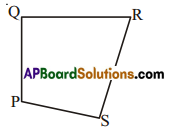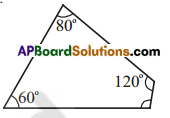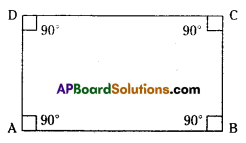AP Board 7th Class Maths Solutions Chapter 12 Quadrilaterals Ex 1

AP State Syllabus AP Board 7th Class Maths Solutions Chapter 12 Quadrilaterals Ex 1 Textbook Questions and Answers.

AP State Syllabus 7th Class Maths Solutions 12th Lesson Quadrilaterals Exercise 1Question 1.
(i) Name the sides, angles, vertices and diagonals.
(ii) Also name all the pairs of adjacent sides, adjacent angles, opposite sides and opposite angles. .Solution:
sides = PQ, QR, RS and SP
angles = ∠P, ∠Q, ∠R, and ∠S
vertices = P, Q, R and S
diagonals = PR and QS
ii) Pairs of adjacent sides = (PQ, QR), (QR. RS), (RS, SP), (SP, PQ)
Pairs of adjacent angles (∠P, ∠Q). (∠Q, ∠R), (∠R, ∠S), (∠S, ∠P)
Pairs of opposite sides = (PQ, RS) and (QR, PS)
Pairs of opposite angles = (∠P,∠R) and (∠Q, ∠S)Question 2.
The three angles of a quadrilateral are 60°, 80° and 1200. Find the fourth angle?
Solution:
Given three angles = 60°,80°,120°
Sum of the given three angles = 60°+ 80° + 120° = 260°
Sum of the four Interior angles of a quadrilateral = 360°
∴ The fourth angle = 360° — 260° = 100°

Question 3.
The angles of a quadrilateral are in the ratio 2 : 3 : 4: 6. Find the measure of each of the four angles.
Solution:Given that ratio of the four angles = 2 : 3 : 4 : 6
Sum of the terms in the ratio = 2 + 3 + 4 + 6 = 15
Sum of the four angles = 360°
∴ 1st angle = $$\frac { 2 }{ 15 }$$ x 360° = 48°
2nd angle = $$\frac { 3 }{ 15 }$$ x 360° = 72°
3rd angle = $$\frac { 4 }{ 15 }$$ x 360° = 96°
4th angle = $$\frac { 6 }{ 15 }$$ x 360° = 144°

Question 4.
Find each of them.
Solution:Sum of the four angles in a quadrilateral = 360°
Each of the angle = $$\frac{360^{\circ}}{4}$$ = 90°
∠A = ∠B = ∠C = ∠D = 90°Question 5.
In a quadrilateral, the angles arex°, (x + l0)°, (i+ 20)°, (x + 30)°. Find the angles.
Solution:
Given angles are x°, (x + 10)°, (x + 20)°, (x + 30)°
Sum of the four angles = x + x + 10° + x + 20° + x + 30° = 4x + 60°
But the sum of the four angles = 360°
4 x 60° = 60° = 360°
4x = 360° – 60° = 300°
x = $$\frac{300^{\circ}}{4}$$ = 75°
∴ The angles are x = 750
x + 10°- 75°+ 10° = 85°
x + 20° = 75° +20° = 95°
x + 30° = 75° + 30° = 105°Question 6.
The angles of a quadrilateral cannot be in the ratio 1: 2 : 3 : 6. Why? Give reasons.
(Hint: Try to draw a rough diagram of this quadrilateral)
Solution:
Given that the angles of a quadrilateral cant be in the ratio 1: 2 : 3 : 6
If the ratio is 1 : 2 : 3 : 6 then
the sum of terms of ratio = I + 2 + 3 + 6 = 12
Sum of the angles = 360°
∴ 1st angle = $$\frac{1}{12}$$ x 360° = 30°
2nd angle = $$\frac{2}{12}$$x 360° = 60°
3rd angle = $$\frac{3}{12}$$ x 360° = 90°
4th angle = $$\frac{3}{12}$$ x 360° = I80
i.e., 4th angle is 180°, a straight angle.
A quadrIlateral cant he formed with these angles.